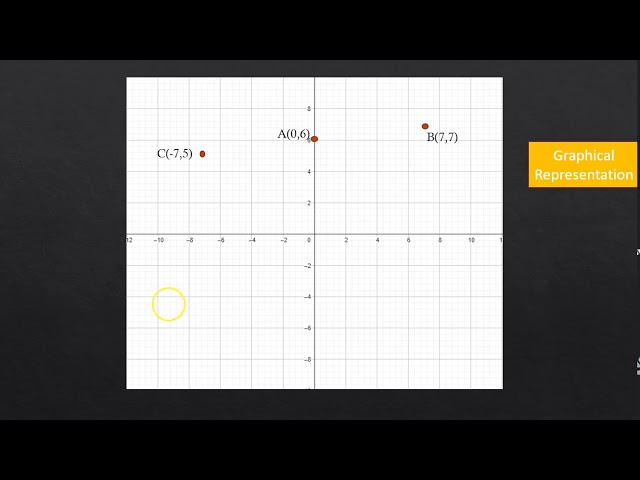# NCERT Solutions for Class 10 Maths Chapter 3 - Pair of Linear Equations in Two Variables

Share

NCERT Mathematics Solutions for Class 10th Chapter 3 - Pair of Linear Equations in Two Variables are provided here by Grade Up School. These Solutions are prepared after extensive research and after considering the latest syllabus prescribed by CBSE in order to help students in covering the complete syllabus of the exam in an efficient way. The step-wise answers to the questions asked in the NCERT textbook assist students in knowing the basic concept of the topics correctly.

NCERT Solutions for Chapter 3- Pair of Linear Equations in Two Variables deals with important topics like Graphical Method of NCERT Solutions of a Pair of Linear Equations, Algebraic Methods of  Solving a Pair of Linear Equations, Substitution Method, Elimination Method, Cross - Multiplication Method and Equations Reducible to a Pair of Linear Equations in Two Variables.

## NCERT Solutions for Class 10 Maths Chapter 3 - Pair of Linear Equations in Two Variables

Exercise 3.1
• Exercise 3.1
• Exercise 3.2
• Exercise 3.3
• Exercise 3.4
• Exercise 3.5
• Exercise 3.6
• Exercise 3.7
Class 10th|NCERT - MathematicsChapter 3 - Pair of Linear Equations in Two VariablesNCERT - MathematicsClass 10th , NCERT
 Chapter 1 - Real Numbers Chapter 2 - Polynomials Chapter 3 - Pair of Linear Equations in Two Variables Chapter 4 - Quadratic Equations Chapter 5 - Arithmetic Progressions Chapter 6 - Triangles Chapter 7 - Coordinate Geometry Chapter 8 - Introduction to Trigonometry Chapter 9 - Some Applications of Trigonometry Chapter 10 - Circles Chapter 11 - Constructions Chapter 12 - Areas Related to Circles Chapter 13 - Surface Areas and Volumes Chapter 14 - Statistics Chapter 15 - Probability